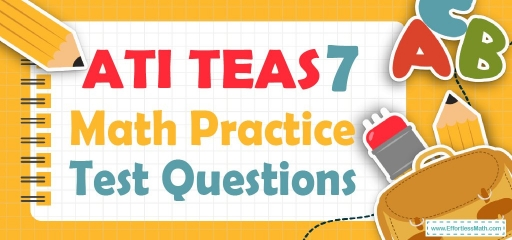# ATI TEAS 7 Math Practice Test QuestionsTaking the ATI TEAS 7 Math test? Try following free ATI TEAS 7 Math Practice questions. Reviewing practice questions is the best way to brush up on your Math skills. Here, we walk you through solving 10 common ATI TEAS 7 Math practice problems covering the most important math concepts on the ATI TEAS 7 Math test.

These ATI TEAS 7 Math practice questions are designed to be similar to those found on the real ATI TEAS 7 Math test. They will assess your level of preparation and will give you a better idea of what to study for your exam.

## 10 Sample ATI TEAS 7 Math Practice Questions

1- The distance between cities A and B is approximately 2,600 miles. If you drive an average of 68 miles per hour, how many hours will it take you to drive from city A to city B?

A. approximately 41 hours

B. approximately 38 hours

C. approximately 29 hours

D. approximately 27 hours

2- If two angles in a triangle measure 53 degrees and 45 degrees, what is the value of the third angle?

A. 8 degrees

B. 42 degrees

C. 98 degrees

D. 82 degrees

3- What is the equivalent temperature of $$104^\circ$$F in Celsius?
$$C = \frac{5}{9} (F -32)$$

A. 32

B. 40

C. 48

D. 52

4- The following pie chart shows the time Jason spent working on his homework last week.
The total time Jason spent on his homework last week was 20 hours.

How much time did Jason spend on History last week?

A. 3.5 hours

B. 5 hours

C. 6.5 hours

D. 7 hours

5- What percentage of the time did Jason spend doing the Writing and English homework?

A. $$23\%$$

B. $$28\%$$

C. $$35\%$$

D. $$25\%$$

6- What’s the next number in the series $${20, 17, 14, 11, ?}$$

A. 6

B. 8

C. 9

D. 15

7- With an $$22\%$$ discount, Ella was able to save $20.42 on a dress. What was the original price of the dress? A.$88.92

B. $90.82 C.$92.82

D. $93.92 8- What is the sum of $$\frac{1}{3}+\frac{2}{5}+\frac{1}{2}$$ ? A. 0.9 B. 1 C. 1.23 D. $$1\frac{7}{30}$$ 9- What is the difference between 864.56 and 631.08? A. 233.84 B. 332.84 C. 233.48 D. 323.48 10- Which of the following is a multiple of 4? A. 38 B. 46 C. 85 D. 104 ## Best ATI TEAS 7 Math Tutor Resource for 2022 ## Answers: 1- B $$Speed = \frac{distance}{time}$$ $$68 = \frac{2,600}{time}→ time = \frac{2,600}{68} = 38.23$$ 2- D $$53 + 45 = 98$$ $$180 – 98 = 82$$ 3- B $$C = \frac{5}{9} (F -32)$$ $$C = \frac{5}{9} (104 -32)=40$$ 4- D $$\frac{35}{100} × 20 = 7$$ 5- B $$13\% +15\% = 28\%$$ 6- B $${20, 17, 14, 11, 8}$$ 7- C $$22\%$$ of $$x = 20.42$$ $$x =\frac{22}{100} x = 20.42$$ $$x = \frac{22 × 20.42}{100}\cong 92.82$$ 8- C $$\frac{1}{3}+\frac{2}{5}+\frac{1}{2}=$$ $$\frac{10+12+15}{30}=\frac{37}{30}=1.23$$ 9- C $$864.56 – 631.08 = 233.48$$ 10- D $$A. \frac{38}{4} = 9.5$$ $$B. \frac{46}{4} = 11.5$$ $$C. \frac{85}{4} = 21.25$$ $$D. \frac{104}{4} = 26$$ Looking for the best resource to help you succeed on the ATI TEAS 7 Math test? ## The Best Books to Ace the ATI TEAS 7 Math Test ## Related to This Article ### More math articles ### What people say about "ATI TEAS 7 Math Practice Test Questions - Effortless Math: We Help Students Learn to LOVE Mathematics"? No one replied yet. X 52% OFF Limited time only! Save Over 52% SAVE$40

It was $76.99 now it is$36.99